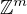Electron. J. Differential Equations, Vol. 2019 (2019), No. 96, pp. 1-14.

### Homoclinic solutions of discrete nonlinear Schrodinger equations with partially sublinear nonlinearities Genghong Lin, Jianshe Yu, Zhan Zhou

Abstract:
We consider a class of discrete nonlinear Schrodinger (DNLS) equations in m dimensional lattices with partially sublinear nonlinearity f. Combining variational methods and a priori estimate, we give a general sufficient condition on f for type (A), that is, a sequence of nontrivial homoclinic solutions accumulating to zero. By using a compact embedding technique, we overcome the loss of compactness due to the problem being set on the unbounded domain. Another obstacle caused by the local definition of f is solved by using the cutoff methods to recover the global property of f. To the best of our knowledge, this is the first time to obtain infinitely many homoclinic solutions for the DNLS equations with partially sublinear nonlinearity. Moreover, we prove that if f is not sublinear, the zero solution is isolated from other homoclinic solutions. Our results show that the sublinearity and oddness of f yield type (A). Without the oddness assumption, we still can prove that this problem has at least a nontrivial homoclinic solution if f is local sublinear, which improves some existing results.

Submitted October 2, 2018. Published August 2, 2019.
Math Subject Classifications: 39A12, 35Q55.
Key Words: Discrete nonlinear Schrodinger equation; discrete breathers; homoclinic solution; partially sublinear nonlinearities; variational method.

Show me the PDF file (343 KB), TEX file for this article.Genghong Lin Center for Applied Mathematics Guangzhou University Guangzhou 510006, China email: lin_genghong@163.com Jianshe Yu Center for Applied Mathematics Guangzhou University Guangzhou 510006, China email: jsyu@gzhu.edu.cn Zhan Zhou Center for Applied Mathematics Guangzhou University Guangzhou 510006, China email: zzhou0321@hotmail.com